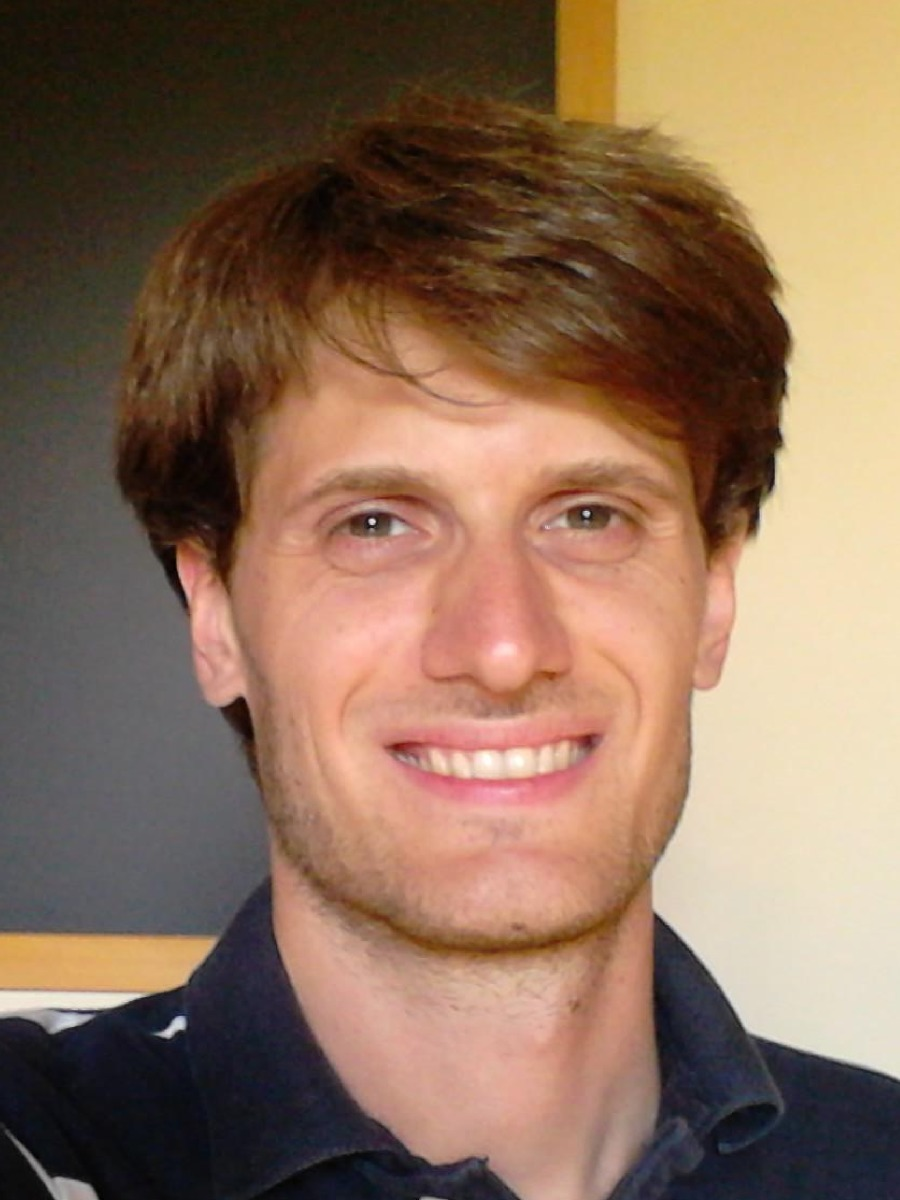# News

## 12/09/2016

Course in San Miniato# Welcome

I am currently Professore Associato (associate professor) in probability and statistics at university of Pisa, department of Mathematics.

Dipartimento di Matematica,
Largo Bruno Pontecorvo 5,
56127 - Pisa (PI) Italy

Here are my contacts:
e-mail dario.trevisan AT unipi.it
office (+39) 050 2213832
mobile (+39) 331 2899761
Skype dario-trevisan# Research

My activity focuses on differential equations, deterministic or stochastic, with low regularity assumptions, both on coefficients and on the ambient space, e.g. on infinite dimensional Gaussian spaces (the framework where Malliavin calculus is developed).

I am also interested in functional inequalities, and their links between probability, geometry and harmonic analysis.

(Picture: superposition of 50 simulated paths of the SDE $dX_t = \mathop{ \rm sign}(X_t) \sqrt{|X_t|} + \varepsilon dB_t$, with $t \in [0,1]$, $X_0=0$, $\varepsilon = 0.01$.)